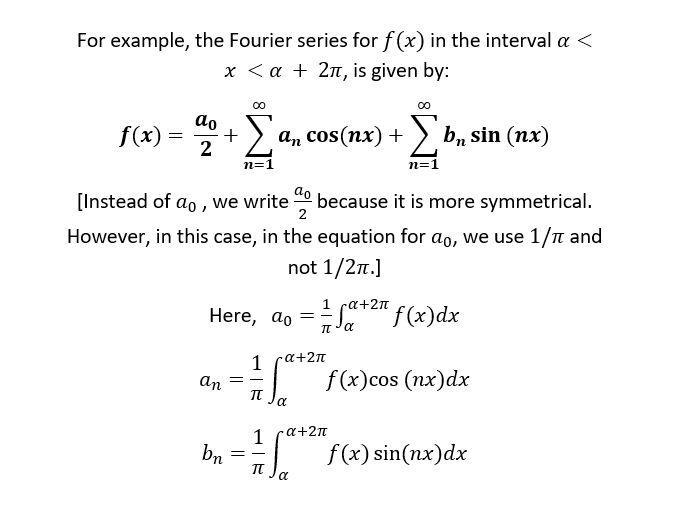top of page
Search

# Is Schrödinger’s Equation Dimensionally Correct? [Review]

Updated: Aug 4

Author: Arpan Dey

Erwin Schrödinger was not happy with Niels Bohr’s old quantum theory. As his background with eigenvalue problems would suggest, he believed that atomic spectra should be determined by some kind of eigenvalue problem. He came up with a revolutionary equation – the famous Schrödinger wave equation.All the equation is telling us is that the Hamiltonian operator, operated on the wave-function, produces energy as a result. The square of the wave-function gives the probability of finding an object in a given region.The Hamiltonian operator has two terms: the first of which represents the kinetic energy, whereas V is the potential energy. The E is the total energy.

The solution of Schrödinger’s equation, the wave-function of the system – can be replaced by an infinite series – the wave-functions of the individual states – which are natural harmonics of each other, i.e., their frequencies are related in the ratio of whole numbers. Here, the concept of Fourier expansion series, a method of solving equations by expressing any mathematical function as the sum of an infinite series of sines and cosines of other periodic functions, comes in.Schrödinger reduced the problem of the energy states in an atom to the problem of finding natural overtones of its vibrating system by using Fourier analysis.

Schrödinger’s equation has been universally recognized as one of the greatest achievements of 20th century science, containing much of physics and in principle, all of chemistry. It is a mathematical tool of power equivalent to the Einstein field equations for gravity, if not more, for dealing with problems of the quantum mechanical model of the atom. This area is generally referred to as wave mechanics.

It is important to note that the wave-function in Schrödinger’s equation spreads out with time. It was widely accepted that matter exhibits both particle and wave properties. However, Schrödinger soon began to suspect this view. He wanted to go back to the classical idea of waves. He started to believe that waves alone can describe everything; particles were useless. Schrödinger proposed that a particle is just a wave group with small dimensions in all directions. However, Hendrik Lorentz pointed out that this wave would spread out with time, and cannot describe particles.

But is the Schrödinger’s equation dimensionally correct? Fundamentally, we know that the effective dimension of the terms in the LHS of a valid equation must be the same as the effective dimension of the terms in the RHS. So yes, of course Schrödinger’s equation is dimensionally correct. But, how do we prove it?Any equation in physics must be dimensionally correct. It would be a great idea to verify this with some other equations.